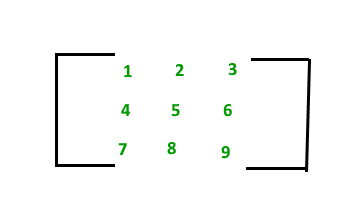# Matrix Data Structure

• Last Updated : 03 Aug, 2023

## What is Matrix Data Structure?

A matrix represents a collection of numbers arranged in an order of rows and columns. It is necessary to enclose the elements of a matrix in parentheses or brackets.
For example:

A matrix with 9 elements is shown below.This Matrix M has 3 rows and 3 columns. Each element of matrix M can be referred to by its row and column number. For example, M = 6.

Topics:

Introduction:

Basic Operations on Matrix:

Standard problems on Matrix: